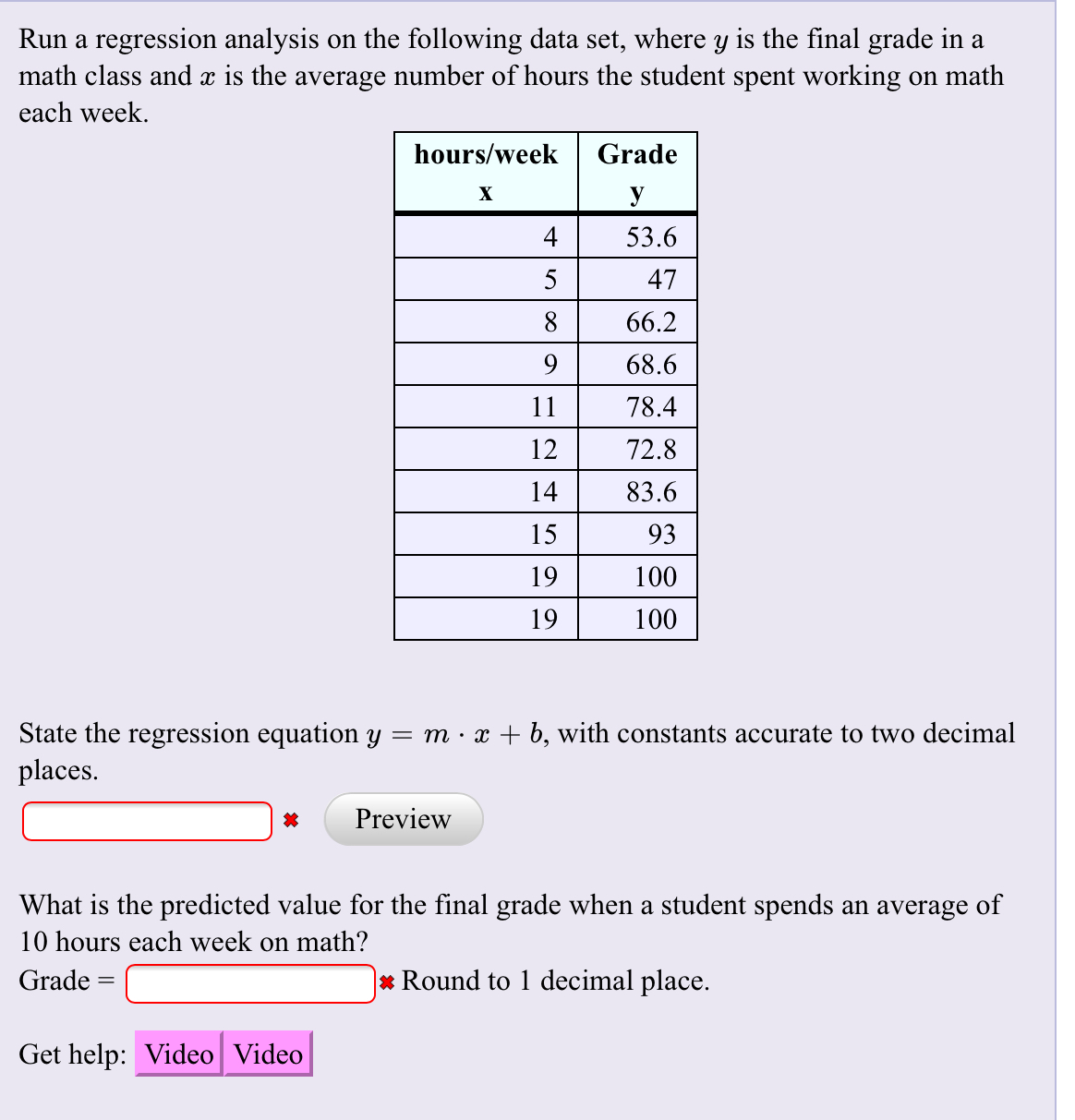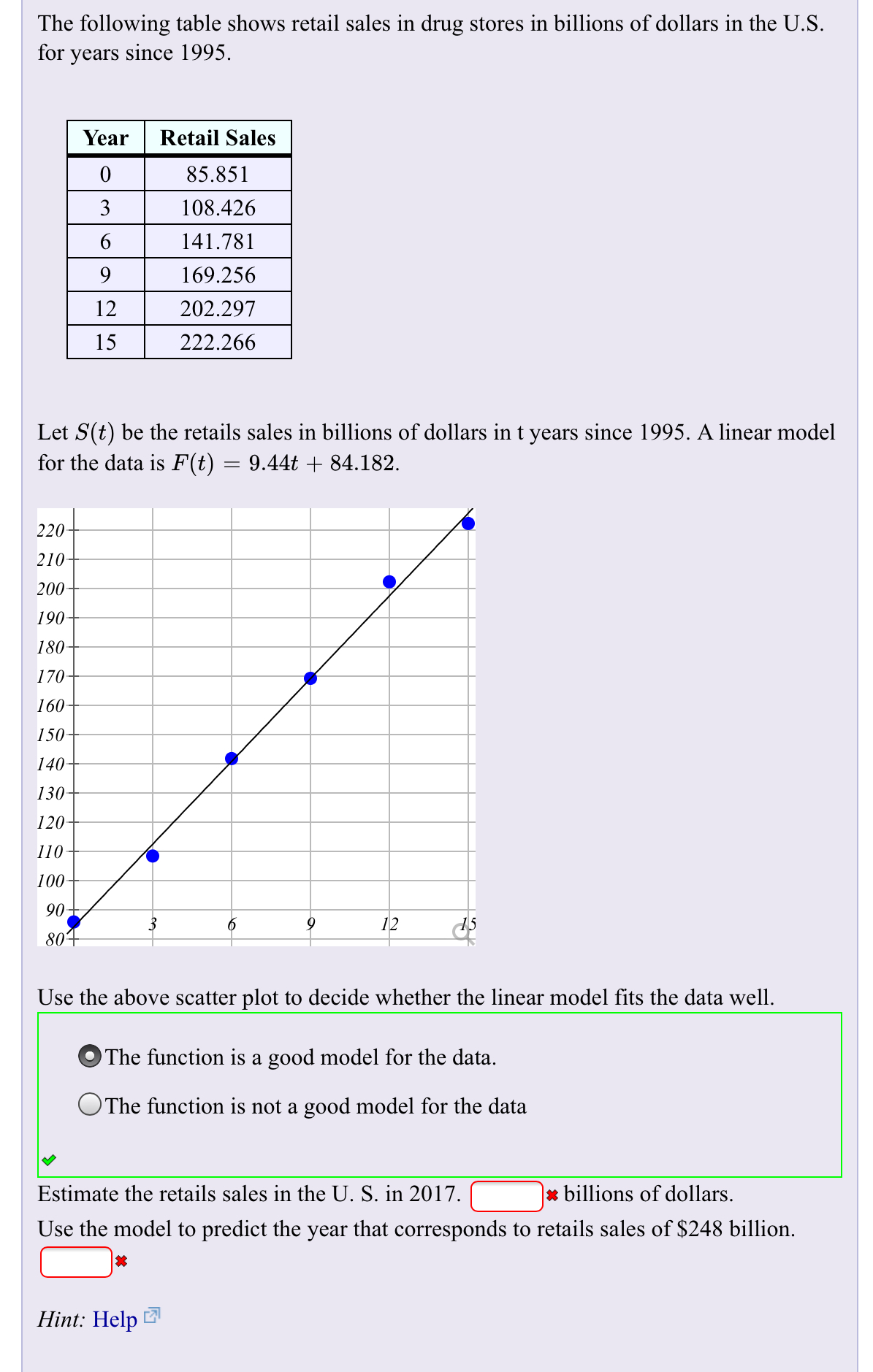# Run a regression analysis on the following data set, where y is the final grade in amath class and x is the average number of hours the student spent working on matheach weekhours/weekGradeXУ53.6547866.2968.61178.41272.81483.615931910019100State the regression equation y = m x + b, with constants accurate to two decimalplaces.Preview*What is the predicted value for the final grade when a student spends an average of10 hours each week on math?* Round to 1 decimal placeGradeGet help: Video Video The following table shows retail sales in drug stores in billions of dollars in the U.Sfor years since 1995YearRetail Sales085.8513108.4266141.7819169.25612202.29715222.266Let S(t) be the retails sales in billions of dollars in t years since 1995. A linear modelfor the data is F(t) = 9.44t + 84.1822202102001901801701601501401301201101009061280Use the above scatter plot to decide whether the linear model fits the data wellThe function is a good model for the data.OThe function is not a good model for the data|x billions of dollarsEstimate the retails sales in the U. S. in 2017.Use the model to predict the year that corresponds to retails sales of \$248 billion.Hint: Help

Question
200 views

I need help finding the answer on the TI84 Plus CE and on paper ?. Thank youhelp_outlineImage TranscriptioncloseRun a regression analysis on the following data set, where y is the final grade in a math class and x is the average number of hours the student spent working on math each week hours/week Grade X У 53.6 5 47 8 66.2 9 68.6 11 78.4 12 72.8 14 83.6 15 93 19 100 19 100 State the regression equation y = m x + b, with constants accurate to two decimal places. Preview * What is the predicted value for the final grade when a student spends an average of 10 hours each week on math? * Round to 1 decimal place Grade Get help: Video Video fullscreenhelp_outlineImage TranscriptioncloseThe following table shows retail sales in drug stores in billions of dollars in the U.S for years since 1995 Year Retail Sales 0 85.851 3 108.426 6 141.781 9 169.256 12 202.297 15 222.266 Let S(t) be the retails sales in billions of dollars in t years since 1995. A linear model for the data is F(t) = 9.44t + 84.182 220 210 200 190 180 170 160 150 140 130 120 110 100 90 6 12 80 Use the above scatter plot to decide whether the linear model fits the data well The function is a good model for the data. OThe function is not a good model for the data |x billions of dollars Estimate the retails sales in the U. S. in 2017. Use the model to predict the year that corresponds to retails sales of \$248 billion. Hint: Help fullscreen
check_circle

Step 1

Note:

Hey there! Thank you for the question. As you have posted two different questions together, according to our policy, ...

### Want to see the full answer?

See Solution

#### Want to see this answer and more?

Solutions are written by subject experts who are available 24/7. Questions are typically answered within 1 hour.*

See Solution
*Response times may vary by subject and question.
Tagged in
MathStatistics

### Other